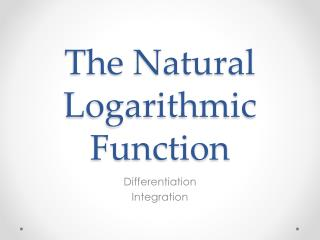Download PresentationThe Natural Logarithmic Function# The Natural Logarithmic Function - PowerPoint PPT Presentation

Download Presentation##### The Natural Logarithmic Function

Download Policy: Content on the Website is provided to you AS IS for your information and personal use and may not be sold / licensed / shared on other websites without getting consent from its author. While downloading, if for some reason you are not able to download a presentation, the publisher may have deleted the file from their server.

- - - - - - - - - - - - - - - - - - - - - - - - - - - E N D - - - - - - - - - - - - - - - - - - - - - - - - - - -
##### Presentation Transcript

1. The Natural Logarithmic Function Differentiation Integration

2. Properties of the Natural Log Function • If a and b are positive numbers and n is rational, then the following properties are true:

3. The Algebra of Logarithmic Expressions

4. The Derivative of the Natural Logarithmic Function Let u be a differentiable function of x

5. Differentiation of Logarithmic Functions

6. Differentiation of Logarithmic Functions

7. Differentiation of Logarithmic Functions

8. Differentiation of Logarithmic Functions

9. Differentiation of Logarithmic Functions

10. Logarithmic Properties as Aids to Differentiation • Differentiate:

11. Logarithmic Properties as Aids to Differentiation • Differentiate:

12. Logarithmic Differentiation • Differentiate: This can get messy with the quotient or product and chain rules. So we will use ln rules to help simplify this and apply implicit differentiation and then we solve for y’…

13. Derivative Involving Absolute Value • Recall that the ln function is undefined for negative numbers, so we often see expressions of the form ln|u|. So the following theorem states that we can differentiate functions of the form y= ln|u| as if the absolute value symbol is not even there. • If u is a differentiable function such that u≠0 then:

14. Derivative Involving Absolute Value • Differentiate:

15. Finding Relative Extrema • Locate the relative extrema of • Differentiate: • Set = 0 to find critical points =0 2x+2=0 X=-1, Plug back into original to find y y=ln(1-2+3)=ln2 So, relative extrema is at (-1, ln2)

16. Homework • 5.1 Natural Logarithmic Functions and the Number e Derivative #19-35,47-65, 71,79,93-96

17. General Power Rule for Integration • Recall that it has an important disclaimer- it doesn’t apply when n = -1. So we can not integrate functions such as f(x)=1/x. • So we use the Second FTC to DEFINE such a function.

18. Integration Formulas • Let u be a differentiable function of x

19. Using the Log Rule for Integration

20. Using the Log Rule with a Change of Variables Let u=4x-1, so du=4dx and dx=

21. Finding Area with the Log Rule • Find the area of the region bounded by the graph of y, the x-axis and the line x=3.

22. Recognizing Quotient Forms of the Log Rule

23. Definition The natural logarithmic function is defined by The domain of the natural logarithmic function is the set of all positive real numbers

24. u-Substitution and the Log Rule

25. Long Division With Integrals

26. How you know it’s long Division • If it is top heavy that means it is long division. • Example

27. Example 1

28. Continue Example 1

29. Example 2

30. Continue Example 2

31. Using Long Division Before Integrating

32. Using a Trigonometric Identity

33. Guidelines for integration • Learn a basic list of integration formulas. (including those given in this section, you now have 12 formulas: the Power Rule, the Log Rule, and ten trigonometric rules. By the end of section 5.7 , this list will have expanded to 20 basic rules) • Find an integration formula that resembles all or part of the integrand, and, by trial and error, find a choice of u that will make the integrand conform to the formula. • If you cannot find a u-substitution that works, try altering the integrand. You might try a trigonometric identity, multiplication and division by the same quantity, or addition and subtraction of the same quantity. Be creative. • If you have access to computer software that will find antiderivatives symbolically, use it.

34. Integrals of the Six Basic Trigonometric Functions

35. Homework • 5.2 Log Rule for Integration and Integrals for Trig Functions (substitution) #1-39, 47-53,  67